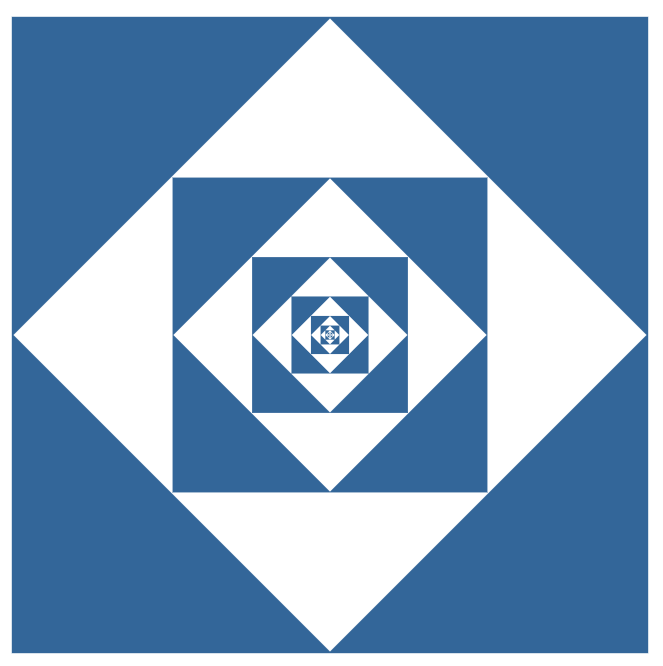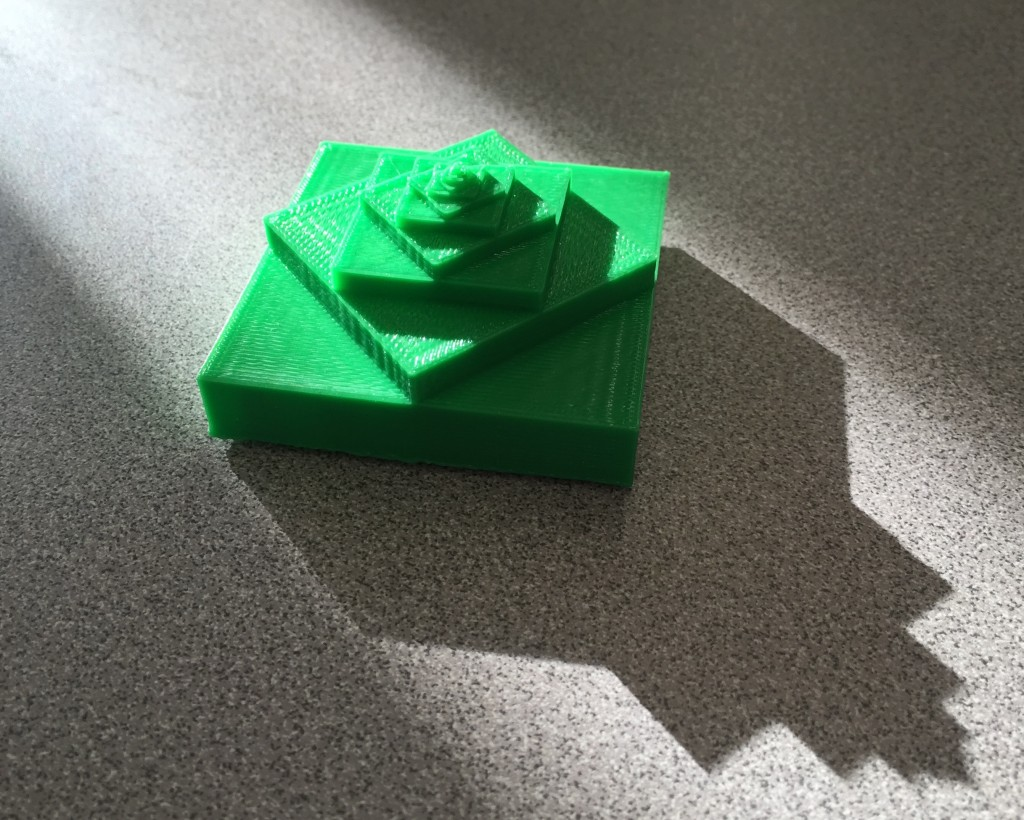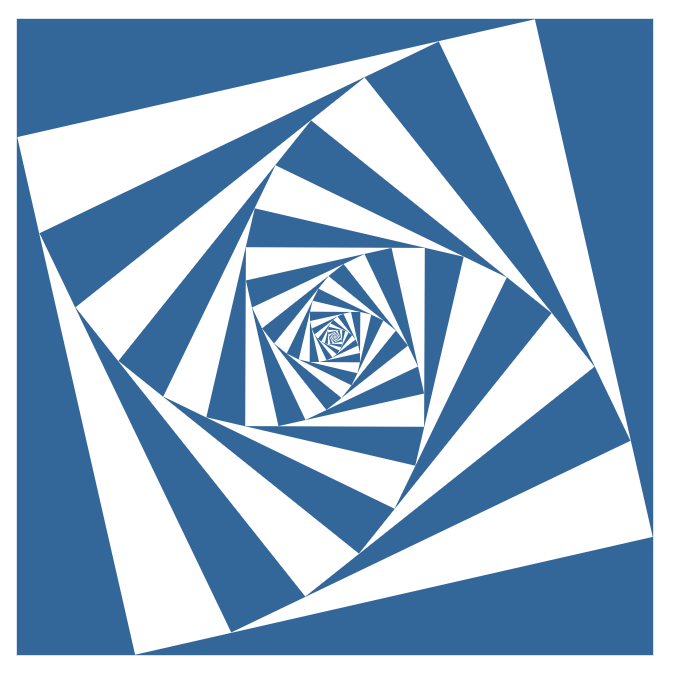# 3d Representation of Dog Chase

Not what it sounds like.
The four dog problem from Steve Strogatz:

## Halfway Dogs

If the dogs blindly travel half way towards the next dog before re-aiming, then you get this picture, where each vertex represents the dog’s position:If you raise each square up in the z-dimension and print the resulting 3d shape then you can get this:## 12% Dogs

If the dogs travel 12% of the way before re-aiming then you get this picture, and this 3d shape:## Interactive 2d Version

The generalized interactive 2d version of this concept is a lot of fun to play with. In this demo, your mouse horizontal controls how much to spin each square, and your mouse vertical controls how much to scale each square. Matt Enlow was the driving force behind this super fun demo. Some samples:

## Code

Here’s the code for both of these shapes in madeup:

### Halfway dogs:

```thickness = 3
size = 10.0
theta = 0
z = 0
repeat 15
t = 0
while t <= 360
x = size * cos (t + theta)
y = size * sin (t + theta)
t = t + 90
moveto x,y,z
end
extrude 0,0,1,thickness
theta = theta + 45
thickness = thickness * (0.5)^0.5
z = z + thickness
size = size * (0.5)^0.5
end
```

### 12% dogs:

```thickness = 1
size = 10.0
theta = 0
z = 0
repeat 35
t = 0
while t <= 360
x = size * cos (t + theta)
y = size * sin (t + theta)
t = t + 90
moveto x,y,z
end
extrude 0,0,1,thickness
theta = theta + 8.775
thickness = thickness * 0.84
z = z + thickness
size = size * 0.8775
end
```
This entry was posted in Full Posts. Bookmark the permalink.